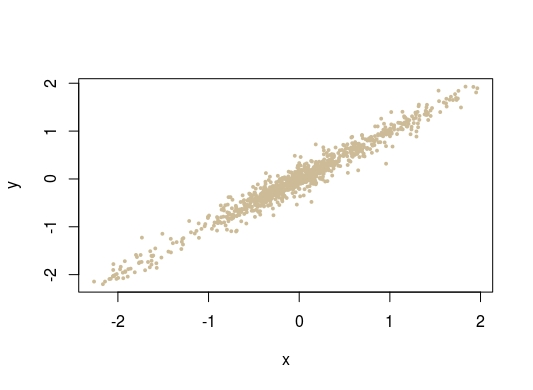Want to share your content on R-bloggers? click here if you have a blog, or here if you don't.

Here are two nice exercises for a future simulation exam, seen and solved on X validated.The first one is about simulating a Gibbs sampler associated with the joint target

exp{-|x|-|y|-a(y-x|}

defined over IR² for a≥0 (or possibly a>-1). The conditionals are identical and non-standard, but a simple bound on the conditional density is the corresponding standard double exponential density, which makes for a straightforward accept-reject implementation. However it is also feasible to break the full conditional into three parts, depending on the respective positions of x, y, and 0, and to obtain easily invertible cdfs on the three intervals.The second exercise is about simulating from the cdf$F(x)=1-\exp\{-ax-bx^{p+1}/(p+1)\}$

which can be numerically inverted. It is however more fun to call for an accept-reject algorithm by bounding the density with a ½ ½ mixture of an Exponential Exp(a) and of the 1/(p+1)-th power of an Exponential Exp(b/(p+1)). Since no extra constant appears in the solution,  I suspect the (p+1) in b/(p+1) was introduced on purpose. As seen in the above fit for 10⁶ simulations (and a=1,b=2,p=3), there is no deviation from the target! There is however an even simpler resolution to the exercise: since the tail function (1-F(x)) appears as the product of two tail functions, exp(-ax) and the other one, the cdf is the distribution of the minimum of two random variates, one with the Exp(a) distribution and the other one being the 1/(p+1)-th power of an Exponential Exp(b/(p+1)) distribution. Which of course returns a very similar histogram fit: